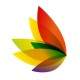# Role of Binary to Decimal in Mathematics and How Can You Learn It Fast

Students who study the fields of computer science, mathematics, and electronics are expected to know the binary system inside out. Along with that, they should also know how binary codes can be converted to decimal, hex and other formats. The binary system is called base 2 while the decimal system is base 10. The reason is that the binary system has two values, 0 and 1. Similarly, the decimal system has values from 0 to 9. Now, when you talk about converting a binary code to the decimal format, certain logics are applied.

## Understanding the binary to decimal logic

Consider the table given below

 32 16 8 4 2 1 1 0 1 1 0 1

• In the above table, the binary code is 101101. Along with that, the decimal values are present in the upper row. Here is how you can determine the decimal form of the binary code 101101.

Decimal form = (1*1) + (0*2) + (1*4) + (1*8) +(0*16) + (1*32)

Decimal Form = 1+0+4+8+0+32

Decimal Form = 45

### Steps for the conversion example

In the above example, the following steps have been performed

1. In the lower row, the binary code has been written and in the upper row, there are certain decimal numbers. If you have a look at the upper row, the numbers increase from right to left. Similarly, they are all a power of 2. For instance, 2 to the power 0 is 1 while 2 to the power 5 has a value of 32. Depending on the binary value you have, the powers of 2 would increase from right to left.
2. Once you have written the corresponding decimal values for the binary code, you can advance to the next step. Simply add the binary numbers with their corresponding decimal numbers and generate a sum. In the above example, this sum is 45. Hence, you can deduce the conclusion that 101101 has a decimal value of 45.

### Learning measures for mathematics students

How do values from one number system get converted to the other? This is an important concept which mathematics students should clearly know about.  These conversion concepts help in understanding a lot of advanced implementations.

• How can a series of 0 and 1 be converted into a single decimal figure. This is exactly what you can learn from this conversion. At the college and undergraduate level, these concepts are taught in a lot of depth. Hence, gaining an understanding about these topics is obviously helpful.

### Get optimum outputs through a binary to decimal converter

There is no doubt that technology has made things a lot easier for the users. One example is the binary to decimal converter by Prepostseo. If you have a look at the example above, you can see that the process is lengthy. If you have to perform more than one conversion in a limited span of time, the difficulty level would obviously increase. An easier option is using the binary to decimal converter.

• This is an automated method to perform this conversion. You can avoid all the conversion steps that have been shown above. These converters are online so you can use them without any downloads.
• When you open the link, enter the input value in the binary form and click the convert button. In few seconds, the output in decimal form would be shown to you.

#### Quickness and accuracy

It is expected that a lot of time would be consumed if you are performing each conversion step manually. As compared to an online converter, the effort would be much more. When you are adhering to strict timelines, manual conversions do not work. Using an online converter gets the job done. Secondly, as a user, you can avoid all kinds of conversion steps. The binary to decimal converter would produce the output for you.

• As humans, we tend to make mistakes particularly when we are performing calculations. During the conversion process even if there is a slight addition error, the final answer would be incorrect. The online converter would not make errors while converting the binary to decimal form. Hence, if you are looking for accurate outputs, using the binary to decimal conversion tool is the best way out.